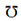## Resistance Assignment Help

Assignment Help: >> Properties of Conductors and Insulators - Resistance

Resistance:

A resistor is the most basic electrical and electronics component that offers a particular amount of electrical resistance to the flow of current while conducted in the circuit.

Resistance is the property of any substance because of which it opposes the flow of current through it and changed electrical energy into heat energy. It has the similar role in electric circuit as that of friction in mechanical system. This opposition is basically because of the molecular structure of the substance. While electrons flow through any substance then they collide with the other molecules or atoms of the substance. In each of the collision, some energy is dissipated in the form of heat. So we may say because of resistance some energy is wasted in the form of heat (which is given by I2 Rt).

The resistance is termed as the ratio of voltage and current in any circuit and its unit is ohm (Ω).

R = V /I ohm (Ω)

Reciprocal of resistance is termed as the conductance (G) and its unit is Siemen mho ().

∴          G = 1/ R = I/ V mho.

On the basis of their resistance, substances can be classified as good conductor, semiconductor and bad conductor.

 Effect of Temperature on Resistance Resistance Law and Resistivity of Material Type of Substances - Basis of their resistance Types of Resistors - On the Basis of Value Voltage and Current Division in Resistive Circuits Voltage and Current Division Rules for Combination of Two Resistances